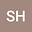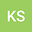p-moment exponential stability of second order differential equations with exponentially distributed moments of impulses
••• Snezhana Hristova,
• Kremena Stefanova
Snezhana Hristova
Plovdiv University
Author ProfileKremena Stefanova
Plovdiv University Paisii Hilendarski
Author Profile## Abstract

Differential equations of second order with impulses at random moments are set up and investigated in this paper. The main characteristic of the studied equations is that the impulses occur at random moments which are exponentially distributed random variables. The presence of random variables in the ordinary differential equation leads to a total change of the behavior of the solution. It is not a function as in the case of deterministic equations, it is a stochastic process. It requires combining of the results in Theory of Differential Equations and Probability Theory. The initial value problem is set up in appropriate way. Sample path solutions are defined as a solutions of ordinary differential equations with determined fixed moments of impulses. P-moment generalized exponential stability is defined and some sufficient conditions for this type of stability are obtained. The study is based on the application of Lyapunov functions. The results are illustrated on example.

#### Peer review status:Published

2021Published in AIMS Mathematics volume 6 issue 3 on pages 2886-2899. 10.3934/math.2021174